# 让人烦恼的初始值

## 通俗易懂但不严谨的解释

“迭代法”也称“辗转法”是一种不断用变量的旧值递推新值的过程。

# 初始值（范围）确定的方法

lrc <- read.csv("data/lrc.csv")

# 光响应曲线比较简单，我们将需要的数据直接提取，方便后面操作
lrc_Q <- lrc$Qin lrc_A <- lrc$A

## nlsLM 解决方案

nlsLM 来自于 Elzhov et al. (2016)minpack.lm，利用 C 语言的 MINPACK 库，修改了 Levenberg-Marquardt 算法，在实际操作中，很多时候并不准确的输入初始值，他也能得出比较好的拟合结果。但结果未必完美，出现下面让人烦恼的报错：

singular gradient matrix at initial parameter estimates

library(minpack.lm)

lrcnls_lm <- nlsLM(lrc_A ~ (1/(2*theta))*
(alpha*lrc_Q+Am-sqrt((alpha*lrc_Q+Am)^2 -
4*alpha*theta*Am*lrc_Q))-
Rd, start=list(Am=(max(lrc_A)-min(lrc_A)),
alpha=0.1,Rd=-min(lrc_A),theta=0.8)) 

summary(lrcnls_lm)
##
## Formula: lrc_A ~ (1/(2 * theta)) * (alpha * lrc_Q + Am - sqrt((alpha *
##     lrc_Q + Am)^2 - 4 * alpha * theta * Am * lrc_Q)) - Rd
##
## Parameters:
##        Estimate Std. Error t value Pr(>|t|)
## Am    12.307570   0.406739  30.259 2.30e-10 ***
## alpha  0.045706   0.003423  13.352 3.09e-07 ***
## Rd     0.656638   0.132646   4.950 0.000791 ***
## theta  0.707522   0.079738   8.873 9.59e-06 ***
## ---
## Signif. codes:  0 '***' 0.001 '**' 0.01 '*' 0.05 '.' 0.1 ' ' 1
##
## Residual standard error: 0.1852 on 9 degrees of freedom
##
## Number of iterations to convergence: 8
## Achieved convergence tolerance: 1.49e-08

### 作图比对法

• Rd、Am等我们可以利用测量值来确定一个范围。
• 剩余的参数，我们也可以根据经验或文献来有一个大致的判断。
• 然后我们根据数学的方式来判断哪个参数对曲线形状影响最大（例如在分母上的参数，或者是乘以该参数，该参数可以显著改变计算结果，例如整体乘以或除以 0.1 还是 0.01，像 Rd 之类的参数本身就很小，多数公式中都是减去该值，对结果影响很小，我们通常直接使用实测值 ）。
• 将该参数取一系列值带入模型来求解净光合速率。
• 将计算的A值与光强进行作图，看我们计算的曲线与测量数据点的重合程度，必要时在修改其他参数，使曲线和散点重合度最好，重合程度最高的参数值即为我们需要的初始值。

### 实现过程

# 我们选择的模型，将其写为一个函数，用于计算净光合速率
expfct <- function(x, Am, alpha, Rd, theta) {(1/(2 * theta)) * (alpha * x + Am - sqrt((alpha * x + Am)^2 - 4 * alpha * theta * Am * x)) - Rd
}

# 我们的数据
test <- data.frame(x = lrc_Q, y = lrc_A)

# 先做实测数据的散点图
plot(y ~ x, data = test)

# 利用上面的函数，假定 alpha 的值为0.8，看计算值与测量值重合程度
curve(expfct(x, Am = (max(lrc_A)-min(lrc_A)),
alpha=0.8, Rd=-min(lrc_A), theta=0.8), add = TRUE
)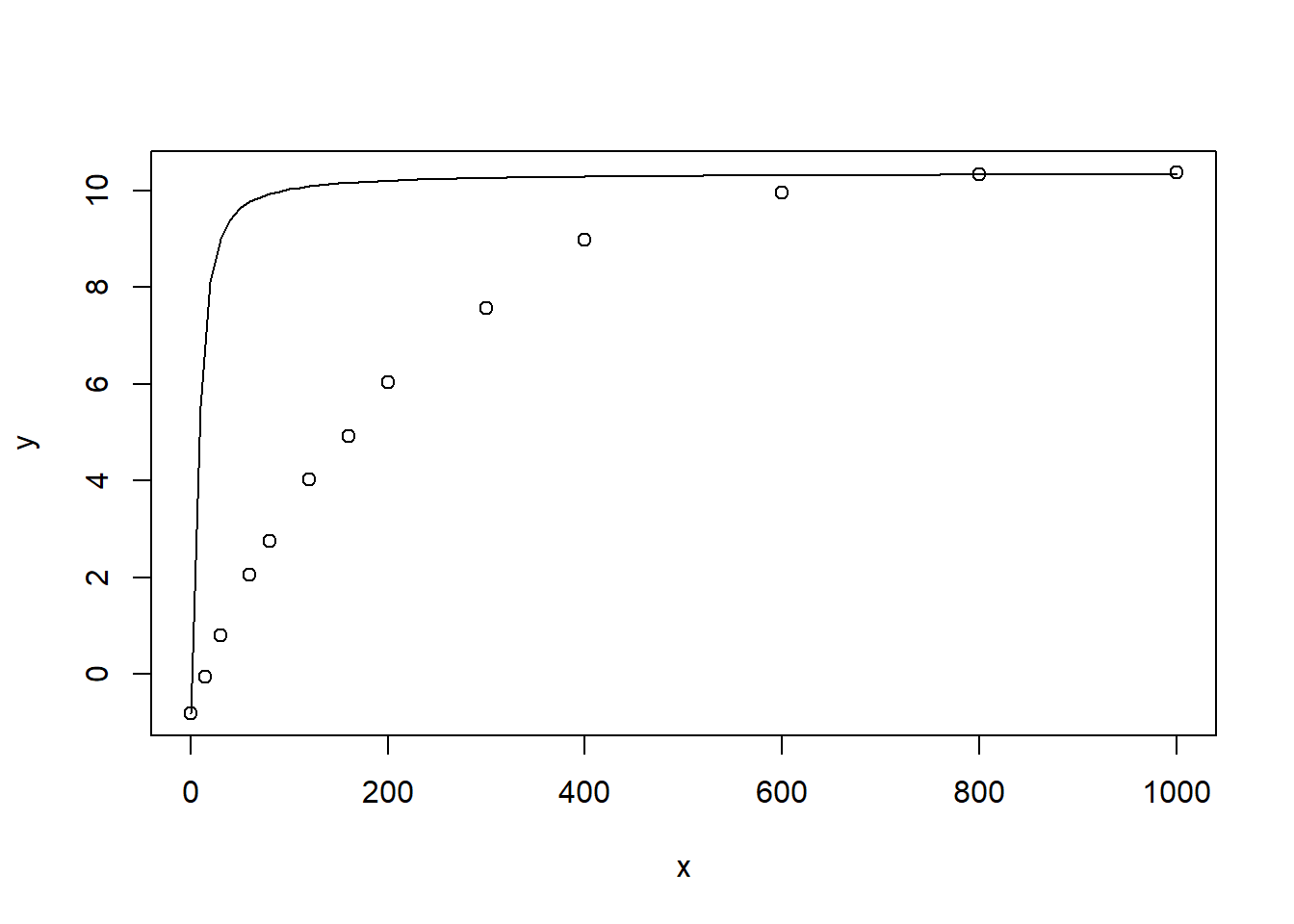plot(y ~ x, data = test)
curve(expfct(x, Am = (max(lrc_A)-min(lrc_A)),
alpha=0.06, Rd=-min(lrc_A), theta=0.82), add = TRUE)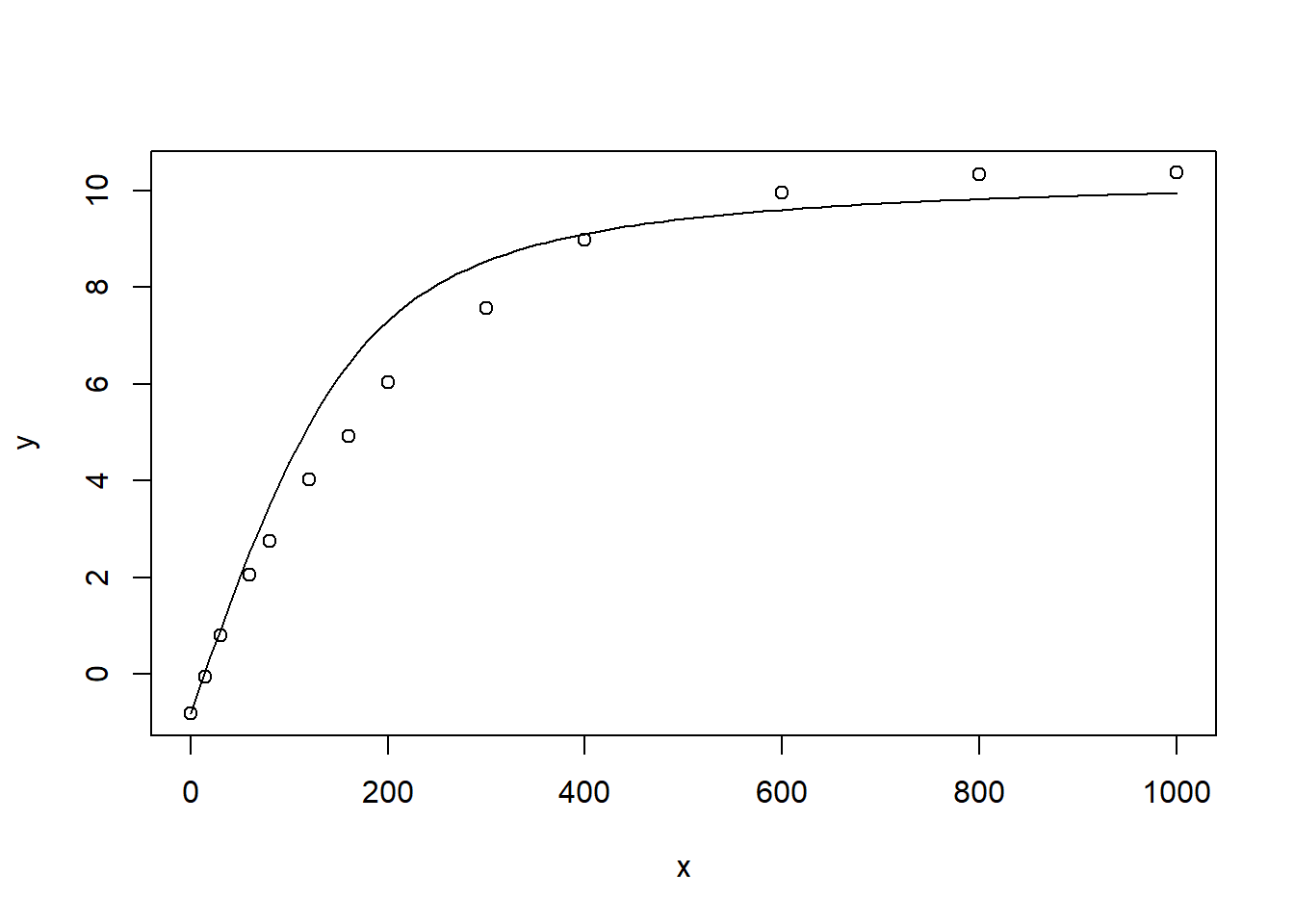lrcnls_manual <- nls(lrc_A ~
(1/(2*theta))*
(alpha*lrc_Q+Am-sqrt((alpha*lrc_Q+Am)^2 -
4*alpha*theta*Am*lrc_Q))-
Rd, start=list(Am=(max(lrc_A)-min(lrc_A)),
alpha=0.03,Rd=-min(lrc_A),theta=0.6))
summary(lrcnls_manual)
##
## Formula: lrc_A ~ (1/(2 * theta)) * (alpha * lrc_Q + Am - sqrt((alpha *
##     lrc_Q + Am)^2 - 4 * alpha * theta * Am * lrc_Q)) - Rd
##
## Parameters:
##        Estimate Std. Error t value Pr(>|t|)
## Am    12.307585   0.406741  30.259 2.30e-10 ***
## alpha  0.045706   0.003423  13.352 3.09e-07 ***
## Rd     0.656642   0.132646   4.950 0.000791 ***
## theta  0.707518   0.079739   8.873 9.59e-06 ***
## ---
## Signif. codes:  0 '***' 0.001 '**' 0.01 '*' 0.05 '.' 0.1 ' ' 1
##
## Residual standard error: 0.1852 on 9 degrees of freedom
##
## Number of iterations to convergence: 7
## Achieved convergence tolerance: 4.601e-06
# 对拟合之后的结果作图，观察使用我们的估计值，
# 迭代的最终值与元数据的重合程度
plot(y ~ x, data = test, ylim = c(-2, 14))
curve(expfct(x, Am = 12.307586,
alpha=0.045706, Rd= 0.656643, theta=0.707518), add = TRUE)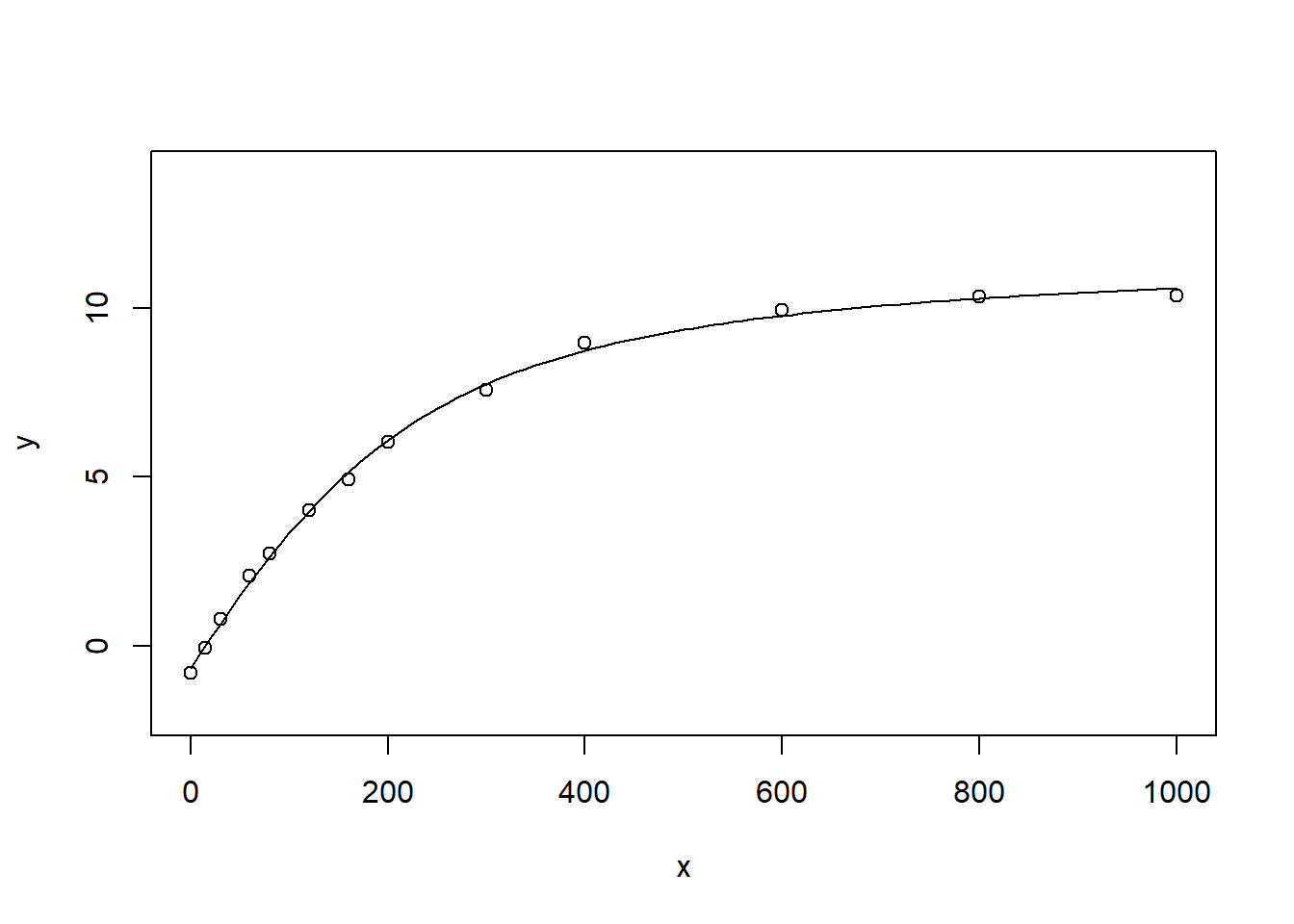### 直观展示

library(ggplot2)
library(purrr)

# 光响应曲线比较简单，我们将需要的数据直接提取，方便后面操作
lrc_Q <- lrc$Qin lrc_A <- lrc$A
n <- length(lrc_A)

alp <- paste0("a=", seq(0.01, 0.07, by = 0.005))
alpn <- rep(alp, each = n)

expfct <- function(x, Am, alpha, Rd, theta) {(1/(2 * theta)) * (alpha * x + Am - sqrt((alpha * x + Am)^2 - 4 * alpha * theta * Am * x)) - Rd
}

paras <- data.frame(alpha = rep(seq(0.01, 0.07, by = 0.005), each = n),
x = rep(lrc_Q, n), Am = rep(12.31, n), Rd = rep(0.66, n),
theta = rep(0.71, n))
y = unlist(pmap(paras, expfct))

show <- data.frame(x = rep(lrc_Q, 14),
y = c(lrc_A, y),
a = factor(c(rep("measured", n), alpn),
level = c("measured", alp)
))

ggplot(data = show, aes(x, y, group = a, color=a)) +
geom_point() +
geom_smooth(se = FALSE)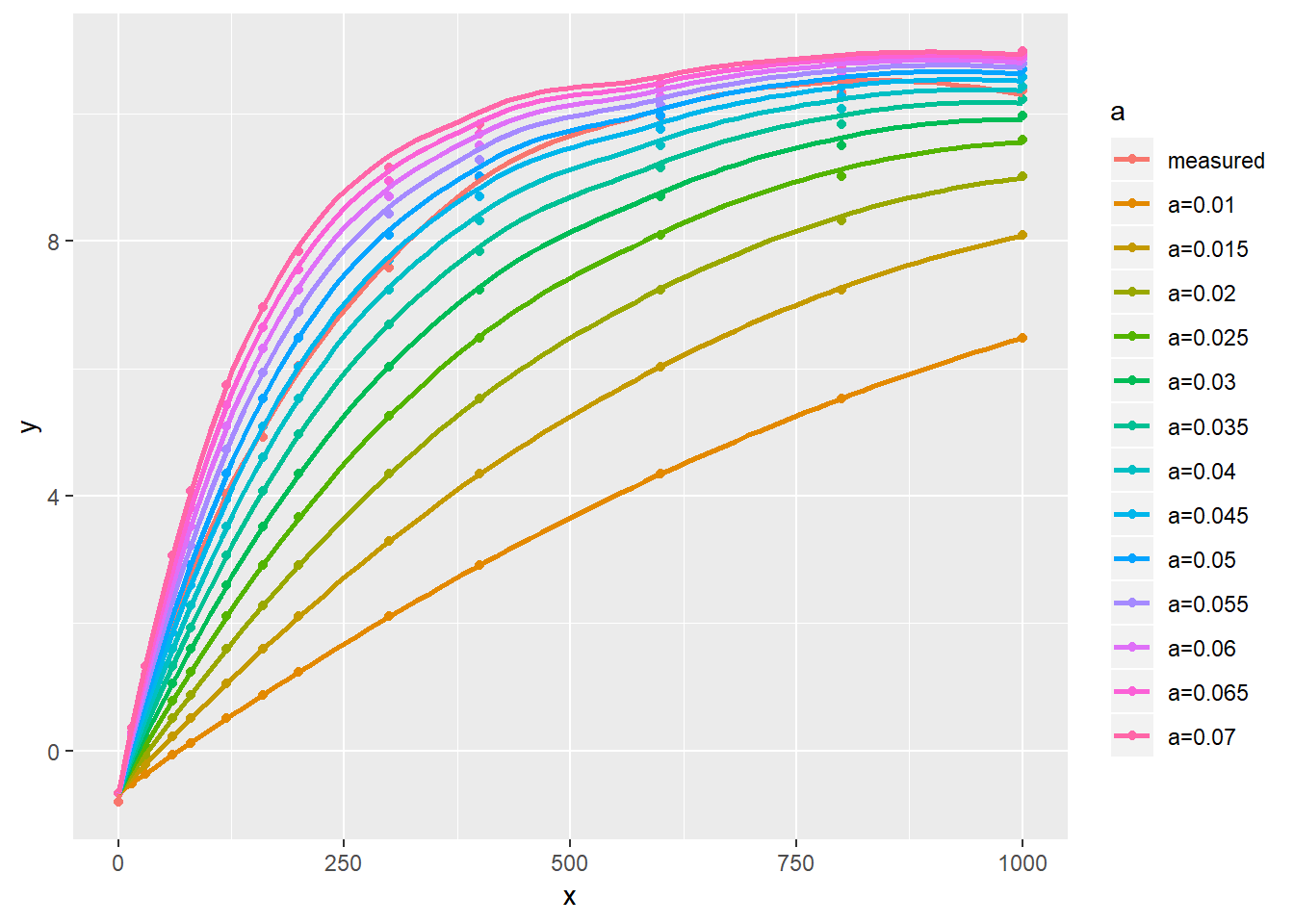## 自动多次尝试法

library(nls2)
## Loading required package: proto
grid.test <- expand.grid(list(
Am=c(12),
alpha = seq(0.01, 0.08, by =0.01),
Rd = seq(0, 3),
theta=seq(0.1, 1, by = 0.1)
))

lrcnls2 <- nls2(lrc_A ~
(1/(2*theta))*
(alpha*lrc_Q+Am-sqrt((alpha*lrc_Q+Am)^2 -
4*alpha*theta*Am*lrc_Q))-
Rd, start = grid.test, algorithm = "brute-force")
summary(lrcnls2)
##
## Formula: lrc_A ~ (1/(2 * theta)) * (alpha * lrc_Q + Am - sqrt((alpha *
##     lrc_Q + Am)^2 - 4 * alpha * theta * Am * lrc_Q)) - Rd
##
## Parameters:
##        Estimate Std. Error t value Pr(>|t|)
## Am    12.000000   0.623023  19.261 1.27e-08 ***
## alpha  0.050000   0.006414   7.795 2.72e-05 ***
## Rd     1.000000   0.260153   3.844  0.00394 **
## theta  0.800000   0.102143   7.832 2.62e-05 ***
## ---
## Signif. codes:  0 '***' 0.001 '**' 0.01 '*' 0.05 '.' 0.1 ' ' 1
##
## Residual standard error: 0.3663 on 9 degrees of freedom
##
## Number of iterations to convergence: 320
## Achieved convergence tolerance: NA

plot(lrc_Q, lrc_A)
lines(lrc_Q,  predict(lrcnls2), col="red")
lines(lrc_Q,  predict(lrcnls_manual), col="blue")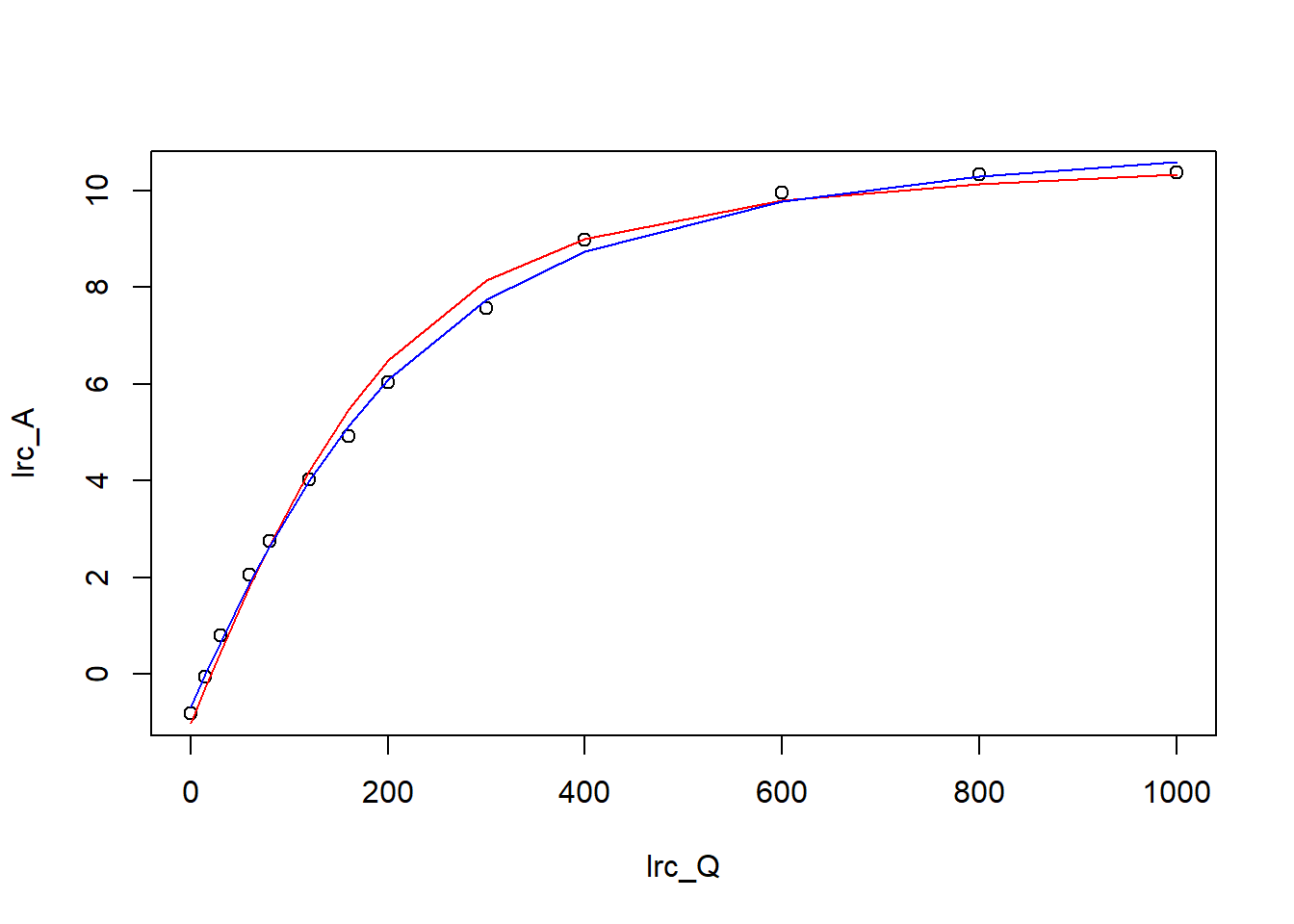# 参考文献

Bouvier A, Huet S. 1994. Nls2 non-linear regression by s-plus—functions. Computational Statistics & Data Analysis 18, 187–190.

Elzhov TV, Mullen KM, Spiess AN, Bolker B. 2016. Minpack.lm: R interface to the levenberg-marquardt nonlinear least-squares algorithm found in minpack, plus support for bounds.

1. 有些时候结果并不理想，该方法并不是万无一失

...

... 本站访问量：   您是本站第 位访问者